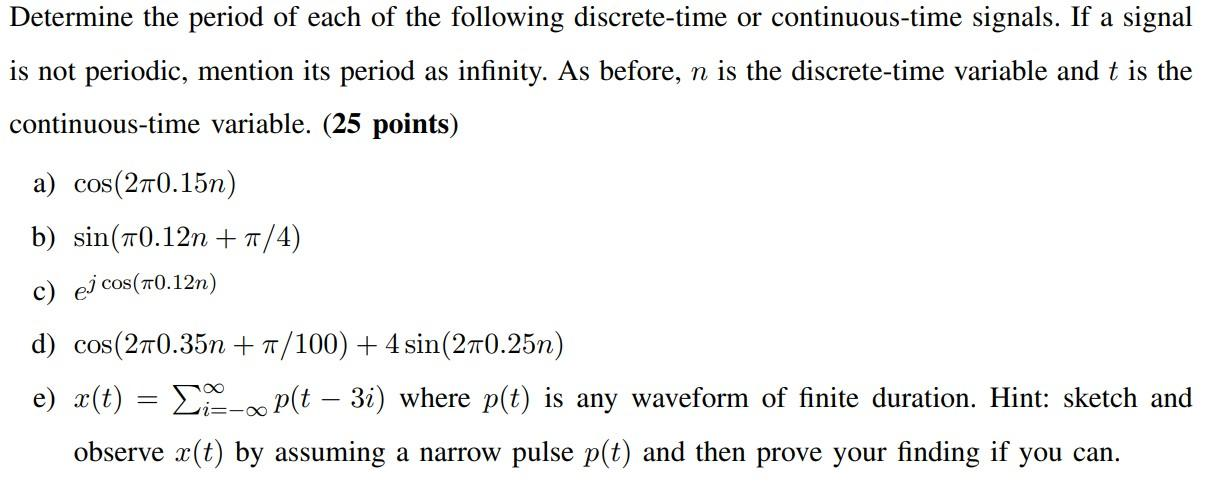# Question Solved1 AnswerDetermine the period of each of the following discrete-time or continuous-time signals. If a signal is not periodic, mention its period as infinity. As before, $$n$$ is the discrete-time variable and $$t$$ is the continuous-time variable. (25 points) a) $$\cos (2 \pi 0.15 n)$$ b) $$\sin (\pi 0.12 n+\pi / 4)$$ c) $$e^{j \cos (\pi 0.12 n)}$$ d) $$\cos (2 \pi 0.35 n+\pi / 100)+4 \sin (2 \pi 0.25 n)$$ e) $$x(t)=\sum_{i=-\infty}^{\infty} p(t-3 i)$$ where $$p(t)$$ is any waveform of finite duration. Hint: sketch and observe $$x(t)$$ by assuming a narrow pulse $$p(t)$$ and then prove your finding if you can.3BUOWS The Asker · Electrical EngineeringTranscribed Image Text: Determine the period of each of the following discrete-time or continuous-time signals. If a signal is not periodic, mention its period as infinity. As before, $$n$$ is the discrete-time variable and $$t$$ is the continuous-time variable. (25 points) a) $$\cos (2 \pi 0.15 n)$$ b) $$\sin (\pi 0.12 n+\pi / 4)$$ c) $$e^{j \cos (\pi 0.12 n)}$$ d) $$\cos (2 \pi 0.35 n+\pi / 100)+4 \sin (2 \pi 0.25 n)$$ e) $$x(t)=\sum_{i=-\infty}^{\infty} p(t-3 i)$$ where $$p(t)$$ is any waveform of finite duration. Hint: sketch and observe $$x(t)$$ by assuming a narrow pulse $$p(t)$$ and then prove your finding if you can.
More
Transcribed Image Text: Determine the period of each of the following discrete-time or continuous-time signals. If a signal is not periodic, mention its period as infinity. As before, $$n$$ is the discrete-time variable and $$t$$ is the continuous-time variable. (25 points) a) $$\cos (2 \pi 0.15 n)$$ b) $$\sin (\pi 0.12 n+\pi / 4)$$ c) $$e^{j \cos (\pi 0.12 n)}$$ d) $$\cos (2 \pi 0.35 n+\pi / 100)+4 \sin (2 \pi 0.25 n)$$ e) $$x(t)=\sum_{i=-\infty}^{\infty} p(t-3 i)$$ where $$p(t)$$ is any waveform of finite duration. Hint: sketch and observe $$x(t)$$ by assuming a narrow pulse $$p(t)$$ and then prove your finding if you can.Step1/2.gkwtCW{margin:0;font-family:"Aspira Webfont","Helvetica","Arial",sans-serif;display:-webkit-box;display:-webkit-flex;display:-ms-flexbox;display:flex;-webkit-flex-direction:column;-ms-flex-direction:column;flex-direction:column;gap:16px;}/*!sc*/data-styled.g379[id="sc-z3f5s1-0"]{content:"gkwtCW,"}/*!sc*/.iIwMoS{white-space:pre-wrap;}/*!sc*/data-styled.g381[id="sc-1aslxm9-0"]{content:"iIwMoS,"}/*!sc*/.fzJtOB{text-align:start;}/*!sc*/data-styled.g383[id="sc-1aslxm9-2"]{content:"fzJtOB,"}/*!sc*/.hOZehF{margin:0;font-family:"Aspira Webfont","Helvetica","Arial",sans-serif;}/*!sc*/data-styled.g410[id="sc-9wsboo-0"]{content:"hOZehF,"}/*!sc*/.lhIoTe{margin:0;font-size:1rem;}/*!sc*/data-styled.g412[id="sc-1swtczx-0"]{content:"lhIoTe,"}/*!sc*/.iHelzO{margin:0;font-family:"Aspira Webfont","Helvetica","Arial",sans-serif;line-height:normal;}/*!sc*/data-styled.g445[id="sc-1sugbjn-0"]{content:"iHelzO,"}/*!sc*/.kkKaFK{margin-top:14px;}/*!sc*/data-styled.g449[id="sc-1sugbjn-4"]{content:"kkKaFK,"}/*!sc*/.iQllJf{margin-top:14px;}/*!sc*/data-styled.g450[id="sc-1sugbjn-5"]{content:"iQllJf,"}/*!sc*/Solution; electrical engineering a) The period of the signal&#160;cos(2&#960;0.15n)&#160;is 1/0.15 = 6.67b) The period of the signal&#160;sin(&#960;0.12n+&#960;/4)&#160;is 1/0.12 = 8.33c) The period of the signal&#160;e^(jcos(&#960;0.12n)) is infinity as it is a complex signal that does not repeat.d) The signal&#160;cos(2&#960;0.35n+&#960;/100)+4sin(2&#960;0.25n)&#160;is a combination of two signals with different periods.&#160;The period of the cosine component is 1/0.35 = 2.86 and the period of the sine component is 1/0.25 = 4.The combined period of the signal is the Least Common Multiple (LCM) of 2.86 and 4.&#160;Since the LCM of two numbers is the smallest number that is divisible by both of them. ... See the full answer